### Ninja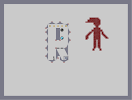Hover over the thumbnail for a full-size version.

Author Nick43092 author:nick43092 rated test 2006-02-09 2006-02-09 2 by 6 people. \$Ninja#Nick43092#none#0000000000000000000000000000000000000000000000000000000000000000000000000000000000000000000000000000000000000000000000000000000000000000000000000000000000000000000000000000000000000000000000000000000000000000000000000000000000000000000GGHGHGHGH0000000000000?000000000@000000000000>000000000A000000000000?011100FJI0000000000000>00000000F@000000000000080000GLHGA00000000000000FIFI00000000000000000000000000000000000000000000000000000000000000000000000000000000000000000000000000000000000000000000000000000000000000000000000000000000000000000000000000000000000000000000000000000000000000000000000000000000000000000000000000000000000000000000000000000000000000000000000000000000000000000000000000000000000000000000000|5^324,348!6^372,228,2,0,0,2!9^396,156,1,0,14,12,1,0,-1!9^300,156,1,0,15,12,1,0,-1!6^372,180,4,0,2,3!0^300,156!0^324,156!0^348,156!0^396,156!0^372,156!12^336,288!12^396,288!12^360,240!12^336,240!12^420,240!12^420,192!12^408,144!12^360,132!12^312,132!12^276,168!12^276,192!12^276,240!12^276,288!12^276,336!12^312,372!12^384,372!11^396,348,396,348!12^348,348!12^348,348!12^564,132!12^576,132!12^576,144!12^576,120!12^588,120!12^588,132!12^588,144!12^600,120!12^600,132!12^600,144!12^564,120!12^552,132!12^540,132!12^552,120!12^564,108!12^576,108!12^588,108!12^564,144!12^588,156!12^588,168!12^576,168!12^576,144!12^576,156!12^516,132!12^528,132!12^528,120!12^540,120!12^552,108!12^540,108!12^588,180!12^576,180!12^564,168!12^564,180!12^552,180!12^552,192!12^600,168!12^600,180!12^612,192!12^600,192!12^612,180!12^564,192!12^588,192!12^576,192!12^612,204!12^624,204!12^624,216!12^636,216!12^648,228!12^636,228!12^600,204!12^600,216!12^600,240!12^600,228!12^588,228!12^564,216!12^564,204!12^576,204!12^588,204!12^588,216!12^576,216!12^576,228!12^588,240!12^588,240!12^576,240!12^540,204!12^540,192!12^528,204!12^528,216!12^516,228!12^516,216!12^528,228!12^576,252!12^588,252!12^600,252!12^564,252!12^564,264!12^576,264!12^588,264!12^600,264!12^564,276!12^528,126!12^522,132!12^534,132!12^534,126!12^540,126!12^546,126!12^546,120!12^534,120!12^546,114!12^540,114!12^558,114!12^570,114!12^564,114!12^552,114!12^546,108!12^558,108!12^570,108!12^582,108!12^546,132!12^552,126!12^558,126!12^558,120!12^576,114!12^588,114!12^582,114!12^576,132!12^570,126!12^570,120!12^564,132!12^564,126!12^582,120!12^582,126!12^582,138!12^594,120!12^594,114!12^600,126!12^588,126!12^576,126!12^594,138!12^588,138!12^600,138!12^594,132!12^582,132!12^570,132!12^570,144!12^570,138!12^564,138!12^582,144!12^576,138!12^594,144!12^582,150!12^582,156!12^582,162!12^576,162!12^588,162!12^576,150!12^588,150!12^570,150!12^594,150!12^558,132!12^582,174!12^570,174!12^600,174!12^594,174!12^594,168!12^594,180!12^588,174!12^582,168!12^582,180!12^576,174!12^570,168!12^564,174!12^564,180!12^570,180!12^558,180!12^606,180!12^612,186!12^606,186!12^600,186!12^594,186!12^588,186!12^582,180!12^582,186!12^576,186!12^564,186!12^558,186!12^570,186!12^552,186!12^546,192!12^564,192!12^540,198!12^546,198!12^534,198!12^534,210!12^528,210!12^522,210!12^534,204!12^522,216!12^522,222!12^516,222!12^528,222!12^522,234!12^516,234!12^522,228!12^570,228!12^570,222!12^570,246!12^570,240!12^570,234!12^576,240!12^576,234!12^582,234!12^588,234!12^594,234!12^600,234!12^588,222!12^600,222!12^588,222!12^594,222!12^588,228!12^594,222!12^594,228!12^582,228!12^582,222!12^576,222!12^642,228!12^630,216!12^618,210!12^624,210!12^630,210!12^630,222!12^636,222!12^642,222!12^618,204!12^618,198!12^612,198!12^618,192!12^600,198!12^588,198!12^594,198!12^594,192!12^588,204!12^594,204!12^582,198!12^582,192!12^582,204!12^576,198!12^570,204!12^570,198!12^576,192!12^564,198!12^570,192!12^564,210!12^564,228!12^564,240!12^564,222!12^564,234!12^570,210!12^576,210!12^582,216!12^570,216!12^582,210!12^588,210!12^594,216!12^594,210!12^600,210!12^576,276!12^588,276!12^600,276!12^594,288!12^594,276!12^594,282!12^588,282!12^600,282!12^594,270!12^594,264!12^594,258!12^594,246!12^570,288!12^570,282!12^570,282!12^564,282!12^570,270!12^576,270!12^564,270!12^570,276!12^570,264!12^570,258!12^570,252!12^576,258!12^576,246!12^570,246!12^564,246!12^564,264!12^564,252!12^564,258!12^588,252!12^594,240!12^600,246!12^588,246!12^588,258!12^600,258!12^600,276!12^588,270!12^600,270!12^594,252!12^594,126!12^600,288!12^564,288!12^576,282!12^600,294!12^606,300!12^606,294!12^600,300!12^564,294!12^564,300!12^558,300!12^558,294# This map is two things, the fourth map in my 'Bye Bye Noob' series, and a dedication to a friend/classmate of mine who just completed N! Enjoy!

## Other maps by this author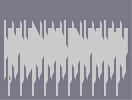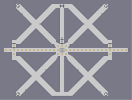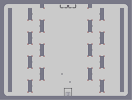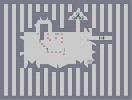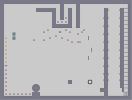Flame Tileset CrossbowTileset Xbow The Third Tower Slants Walk the Walk